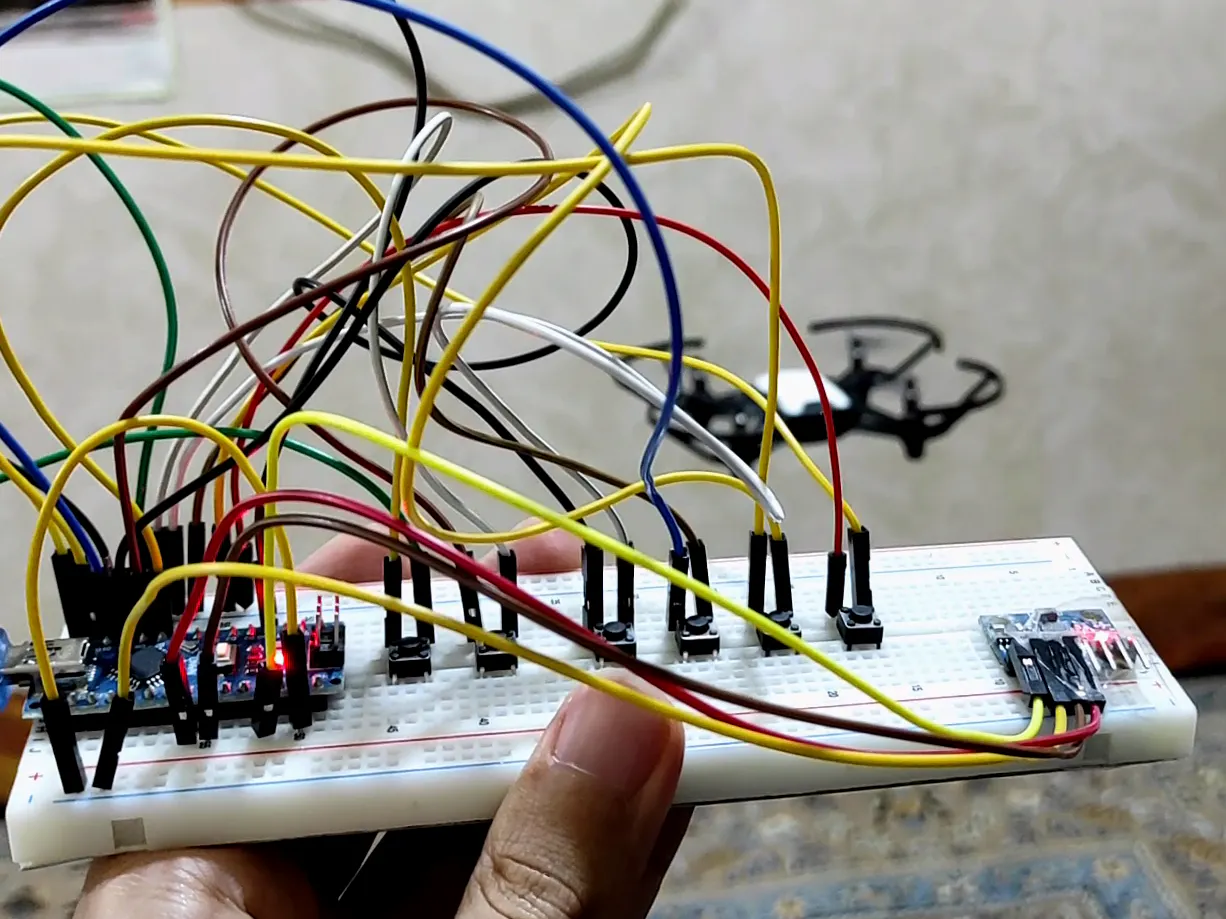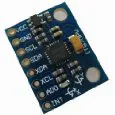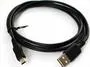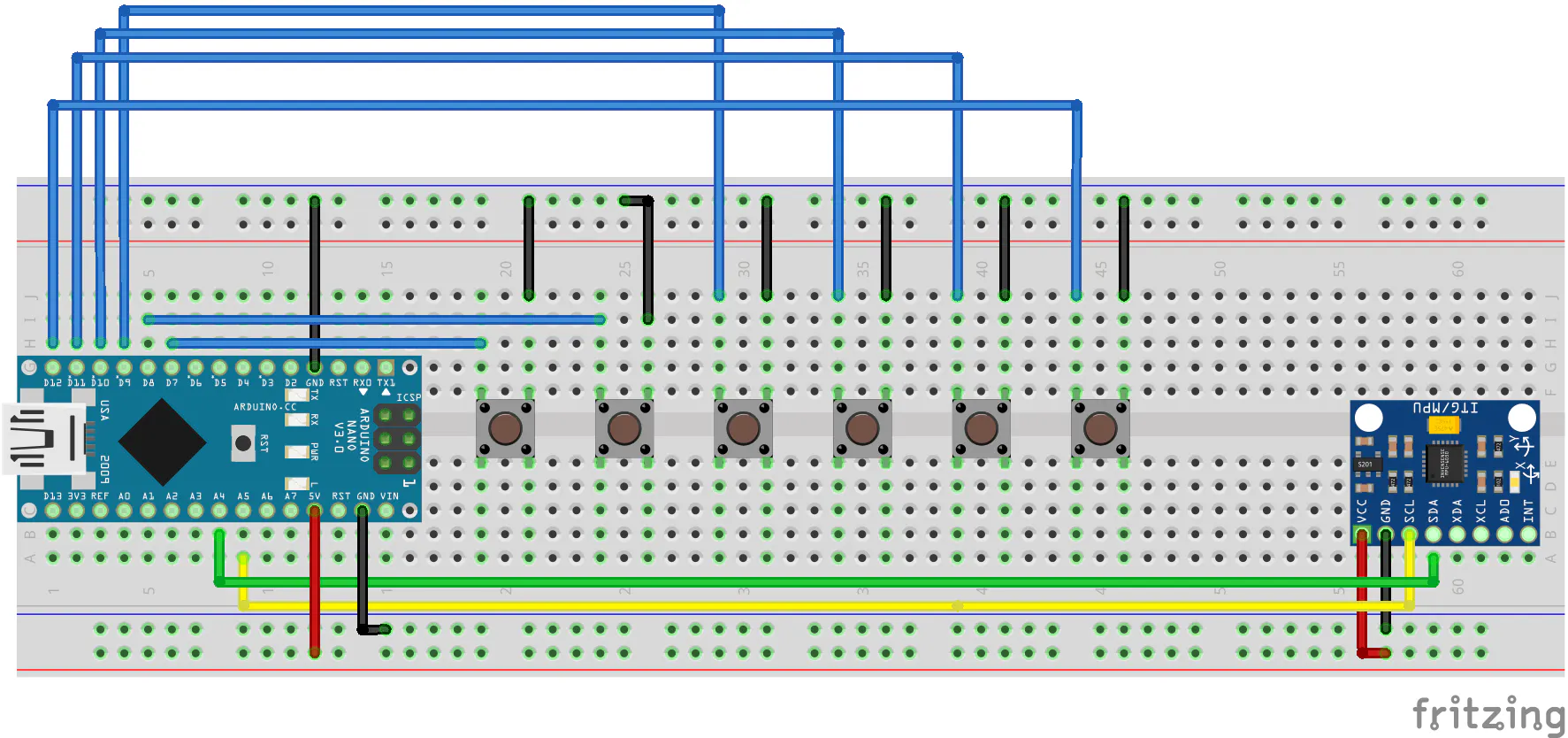How to make a Controller for DJI Tello Drone using Arduino

In this tutorial we learn how to build a DIY Controller for DJI Tello Drone using an Arduino and MPU-6050.

IntermediateFull instructions provided5 hours52Things used in this project

Hardware componentsArduino Nano R3
×1Inertial Measurement Unit (IMU) (6 deg of freedom)
×1SparkFun Pushbutton switch 12mm
×6Jumper wires (generic)
×20USB-A to Mini-USB Cable
×1

Schematics

Controller Circuit DiagramCode

Arduino source code.

C/C++
You must upload this code on Arduino.
#include <Wire.h>

int TakeoffBtn = 7;
boolean TakeoffState;

int LandingBtn = 8;
boolean LandingState;

int UpBtn = 9;
boolean UpState;

int DownBtn = 10;
boolean DownState;

int cwBtn = 11;
boolean cwState;

int ccwBtn = 12;
boolean ccwState;

int ImageBtn = 6;
boolean image;

int Xacceleration;
int Yacceleration;

void setup() {
pinMode(TakeoffBtn,INPUT_PULLUP);
pinMode(LandingBtn,INPUT_PULLUP);
pinMode(UpBtn,INPUT_PULLUP);
pinMode(DownBtn,INPUT_PULLUP);
pinMode(cwBtn,INPUT_PULLUP);
pinMode(ccwBtn,INPUT_PULLUP);
pinMode(ImageBtn,INPUT_PULLUP);

TakeoffState = HIGH;
LandingState = HIGH;
UpState = HIGH;
DownState = HIGH;
cwState = HIGH;
ccwState = HIGH;
image = HIGH;

Serial.begin(115200);
Serial.setTimeout(1);

while (!Serial)
delay(10); // will pause Zero, Leonardo, etc until serial console opens

// Try to initialize!
if (!mpu.begin()) {
Serial.println("Failed to find MPU6050 chip");
while (1) {
delay(10);
}
}
Serial.println("MPU6050 Found!");

mpu.setAccelerometerRange(MPU6050_RANGE_8_G);
Serial.print("Accelerometer range set to: ");
switch (mpu.getAccelerometerRange()) {
case MPU6050_RANGE_2_G:
Serial.println("+-2G");
break;
case MPU6050_RANGE_4_G:
Serial.println("+-4G");
break;
case MPU6050_RANGE_8_G:
Serial.println("+-8G");
break;
case MPU6050_RANGE_16_G:
Serial.println("+-16G");
break;
}
mpu.setGyroRange(MPU6050_RANGE_500_DEG);
Serial.print("Gyro range set to: ");
switch (mpu.getGyroRange()) {
case MPU6050_RANGE_250_DEG:
Serial.println("+- 250 deg/s");
break;
case MPU6050_RANGE_500_DEG:
Serial.println("+- 500 deg/s");
break;
case MPU6050_RANGE_1000_DEG:
Serial.println("+- 1000 deg/s");
break;
case MPU6050_RANGE_2000_DEG:
Serial.println("+- 2000 deg/s");
break;
}

mpu.setFilterBandwidth(MPU6050_BAND_21_HZ);
Serial.print("Filter bandwidth set to: ");
switch (mpu.getFilterBandwidth()) {
case MPU6050_BAND_260_HZ:
Serial.println("260 Hz");
break;
case MPU6050_BAND_184_HZ:
Serial.println("184 Hz");
break;
case MPU6050_BAND_94_HZ:
Serial.println("94 Hz");
break;
case MPU6050_BAND_44_HZ:
Serial.println("44 Hz");
break;
case MPU6050_BAND_21_HZ:
Serial.println("21 Hz");
break;
case MPU6050_BAND_10_HZ:
Serial.println("10 Hz");
break;
case MPU6050_BAND_5_HZ:
Serial.println("5 Hz");
break;
}

Serial.println("");
delay(100);

}
void loop() {

/* Get new sensor events with the readings */

sensors_event_t a, g, temp;
mpu.getEvent(&a, &g, &temp);

Xacceleration = a.acceleration.x;
Yacceleration = a.acceleration.y;

/*
Serial.print("X= ");
Serial.println(Xacceleration);
Serial.print("Y= ");
Serial.println(Yacceleration);
Serial.println("---------------------");
delay(1000);
*/

if((Xacceleration > 6) && (Yacceleration == 0)){
Serial.println("b");
delay(1000);
}
else if((Yacceleration <= 0) && (Xacceleration < -6)){
Serial.println("f");
delay(1000);
}
else if((Xacceleration == 0) && (Yacceleration < -6)){
Serial.println("l");
delay(1000);
}
else if((Xacceleration == 0) && (Yacceleration > 6)){
Serial.println("r");
delay(1000);
}
else if(TakeoffState == LOW){
Serial.println("t");
delay(7000);
}
else if(LandingState == LOW){
Serial.println("c");
delay(7000);
}
else if(UpState == LOW){
Serial.println("u");
delay(1000);
}
else if(DownState == LOW){
Serial.println("d");
delay(1000);
}
else if(cwState == LOW){
Serial.println("p");
delay(1000);
}
else if(ccwState == LOW){
Serial.println("o");
delay(1000);
}
else if(image == LOW){
Serial.println("i");
delay(1000);
}
else{
Serial.println("");
}

}

Python script for Controlling DJI Tello Drone.

Python
After connecting the computer WiFi to the quadcopter. Run this program.
import easytello as tello
import serial
import time

arduino = serial.Serial(port='COM10', baudrate=115200, timeout=.1)

drone = tello.Tello()

while True:

print(cmd)
if cmd == b't\r\n':
drone.takeoff()
elif cmd == b'c\r\n':
drone.land()
elif cmd == b'f\r\n':
drone.forward(40)
elif cmd == b'b\r\n':
drone.back(40)
elif cmd == b'r\r\n':
drone.right(40)
elif cmd == b'l\r\n':
drone.left(40)
elif cmd == b'u\r\n':
drone.up(40)
elif cmd == b'd\r\n':
drone.down(40)
elif cmd == b'p\r\n':
drone.cw(90)
elif cmd == b'o\r\n':
drone.ccw(90)
else:
pass

My Github page

You can see all details in my Github page.